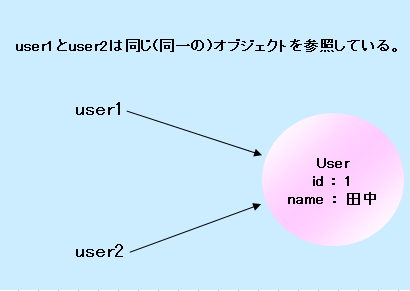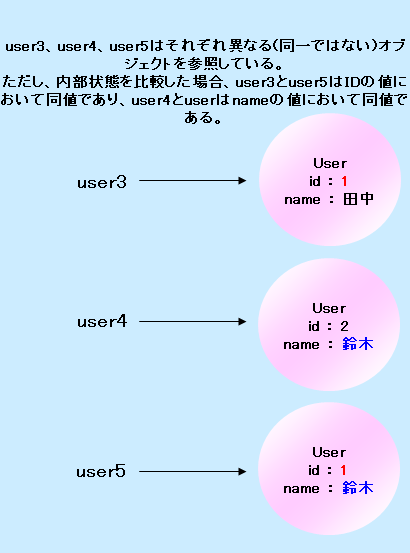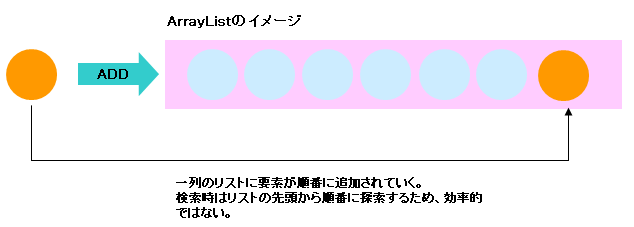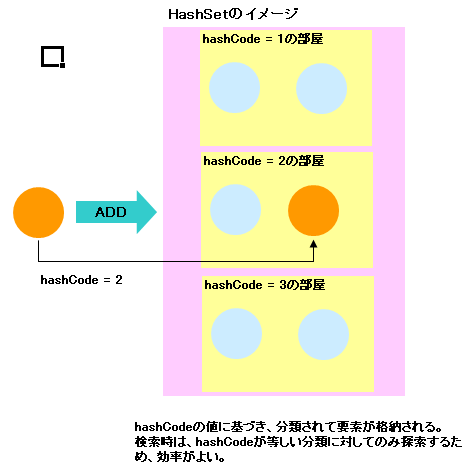# Understanding equals and hashCode in Java

This document is a reprint of the material created for new engineers about 10 years ago. Some old-fashioned parts such as API and syntax may be noticeable, but once you learn the idea, I intend to organize the basic parts that can be used for a long time, so I hope that it will be helpful for young engineers now.

# Point of understanding

--Understand the difference between the equality operator "==" and the equals method in Java --Understanding how to implement equals methods in Java --Understand the meaning of the hashCode method in Java

# Prerequisites

Assuming that the User class exists as shown below, read on. The User class holds its ID and name as an internal state.

#### `User.java`

``````
class User {

/** ID */
private int id;

/**name*/
private String name;

/**
*It is a constructor.
*
* @param id ID
* @param name name
*/
public User(int id, String name) {

this.id = id;
this.name = name;
}

//Omitted below
}
``````

# Difference between identity and equivalence

## What is the "identity" of an object?

``````    User user1 = new User(1, "Tanaka");
User user2 = user1;
``````user1 and user2 hold a reference to the same object. Therefore, the objects referenced by user1 and user2 are "identical".

## What is the "equivalence" of an object?

``````    User user3 = new User(1, "Tanaka");
User user4 = new User(2, "Suzuki");
User user5 = new User(1, "Suzuki");
``````user3 and user5 have the same ID. user4 and user5 have the same name.

Therefore, user3 and user5 are objects that are "equivalent" in ID value. user4 and user5 are objects that are "equivalent" in their name values.

Object equivalence must be implemented by the programmer himself according to business rules.

The equivalence of objects is defined in the equals method of the corresponding class.

If there is a rule to judge the equivalence of the object of User class in the ID value, describe the process to compare the ID value in the equals method.

If there is a rule to judge the equivalence of the object of User class in the name value, describe the process to compare the name value in the equals method.

## How to determine the identity of an object

Object identity is determined by the comparison operator "==". Since user1 and user2 above are the same object

``````    user1 == user2 // ⇒ true
``````

The result of is "true".

Because user3, user4 and user5 are all different objects

``````    user3 == user4 // ⇒ false
user3 == user5 // ⇒ false
user4 == user5 // ⇒ false
``````

The result of is all "false".

## How to determine the equivalence of objects

The equivalence of objects is compared by the "equals" method implemented in each class.

If the equals method of the User class is implemented with ID as the comparison condition, the result of the equals method is as follows.

``````    user3.equals(user4) // ⇒ false
user3.equals(user5) // ⇒ true
user4.equals(user5) // ⇒ false
``````

If the equals method of the User class is implemented with the name as the comparison condition, the result of the equals method is as follows.

``````    user3.equals(user4) // ⇒ false
user3.equals(user5) // ⇒ false
user4.equals(user5) // ⇒ true
``````

# Implementation of equals method

## Implementation example of equals method by user ID

If you want to judge the object equivalence of User class by ID, implement equals method in User class as follows.

#### `User.java`

``````
/**
*The instance of this class and the object passed as an argument
*Returns true if they are equivalent.
*The object passed as an argument is an instance of User class
*If the id values are equal, they are considered equivalent.
*
* @True if the object passed in the return argument is an instance of the User class and the ids are equal.
*/
public boolean equals(Object other) {

if (this == other) { //True if the object passed as an argument is this object itself
return true;
}

if (!(other instanceof User)) { //The object passed as an argument is an object of User class
return false;               //False if not.
}

User otherUser = (User) other;
if (this.id == otherUser.getId()) { //Compare the ID values, true if they are equal, false if they are not equal.
return true;
}
return false;
}
``````

## Implementation example of equals method by user name (name)

To determine the object equivalence of the User class by name, implement the equals method in the User class as follows.

The rest of the process is the same, only the value comparison changes from id to name.

#### `User.java`

``````
/**
*The instance of this class and the object passed as an argument
*Returns true if they are equivalent.
*The object passed as an argument is an instance of User class
*If the values of name are equal, they are considered equivalent.
*
* @True if the object passed in the return argument is an instance of the User class and the names are equal.
*/
public boolean equals(Object other) {

if (this == other) {
return true;
}

if (!(other instanceof User)) {
return false;
}

User otherUser = (User) other;
if (this.name.equals(otherUser.getName())) {
return true;
}
return false;
}
``````

# Understand the hashCode method

## What is hashCode

If you implement the equals method, you must also implement the hashCode method. The hashCode method is implemented according to the following rules.

--Objects for which the result of the equals method is true must return the same value as the result of calling the hashCode method. (It doesn't matter if the object whose equals is false returns the same hashCode result)

In the User class, when the equals method that determines equivalence by the ID value is implemented, the hashCode method is implemented as follows as an example.

### Example 1

#### `User.java`

``````
/**
*Returns the hash code.
*
* @return Hash value of an instance of this class
*/
public int hashCode() {
return this.id;
}
``````

In the equals method, equivalence is judged based on the ID value, so the hashCode method of the object whose ID values are equal, that is, the equals method returns true, will return the same value by the above implementation. , It can be said that the processing of the hashCode method above is valid for the above rule.

### Example 2

#### `User.java`

``````
/**
*Returns the hash code.
*
* @return Hash value of an instance of this class
*/
public int hashCode() {
return 0;
}
``````

The processing of the hashCode method above is actually valid. The hashCode for all objects returns 0. In other words, all objects for which the equals method is true will return the same value (0) as the return value of hashCode. Since it is okay for objects whose equals method is not true (the result of calling the equals method is false) to return the same hashCode method, the above implementation is also considered okay in light of the rules of the hashCode implementation. ..

However, the implementation in Example 2 is not recommended. When implementing hashCode, please implement at least as in Example 1.

## If you did not implement hashCode correctly

If you add an object that does not implement hashCode correctly to an instance of a class that uses a hash algorithm (HashMap, HashSet, etc.), the expected behavior will not be obtained.

## What is a hash algorithm?

It is an algorithm that streamlines processing by calculating the hash value in advance when storing and searching for objects, and storing and searching for objects based on the obtained hash value.

To understand the hash algorithm, it's a good idea to compare the differences in behavior between ArrayList and HashSet.

ArrayList and HashSet are both classes that implement the Collection interface, but there are the following differences in behavior. (HashSet implements a hash algorithm.)

### For ArrayListArrayList stores and holds the added elements in a one-column list. When retrieving the stored elements, the equals method of the object is called in order from the beginning of the list, and the element for which the result of the equals method call is true is returned as the return value.

⇒ If the number of elements in the list is huge and the element you want to retrieve exists at the end of the list, the search efficiency may be extremely deteriorated.

### For HashSetHashSet stores and searches the added elements according to the following procedure.

1. Call the hashCode method of the object to be added.
2. Classify the object into "room" (the implementation term of the HashSet class is Bucket) for each return value of the hashCode method, and store the target object in the "room" with the room number of hashCode.
3. When extracting an element, first search for the target object in the "room" that has the hashCode value as the room number based on the hashCode value.
4. Call the equals method in order for the objects stored in the "room" found in step 3, and return the element for which the result of the equals method call is true as the return value.

Since the elements are classified into "rooms" based on hashCode in advance and stored, when comparing objects, it is only necessary to compare a limited number of objects, which improves search efficiency.

If hashCode is not implemented correctly, it may happen that the target object cannot be found because the target object is searched for in a different room even though the objects have the same value.

If hashCode is implemented to return 0, all objects will be stored in the "room" with the actual room number 0, and the algorithm will be the same as adding an element to the ArrayList, which has the advantage of the hash algorithm. I can't get it.

# The identity of equals and hashCode

## If you did not implement the equals and hashCode methods

The equals and hashCode methods are originally implemented in the Object class.

The Object class is a superclass of all classes. Therefore, if you call the equals method or hashCode method on an instance of a class that does not implement the equals method and hashCode method, it will be defined in the parent class Object class (if there is no other inheriting class). The equals method and hashCode method are called.

For example, when the equals method is called for an instance of a class that does not implement the equals method, the method call order is as follows.

1. The equals method is called.
2. Since the equals method is not defined in the class of the corresponding instance, search retroactively if it is not defined in the parent class.
3. Go back to the parent class, and if any class defines an equals method, that equals method will be called.
4. If you go back to the parent class and no equals method is defined in any of the classes, you will finally reach the definition of the Object class and the equals method defined in the Object class will be called.

## What it means to implement the equals and hashCode methods

"Implementing equals method and hashCode method" means (when there is no other class to inherit)

--"Change the behavior of the equals method and hashCode method of the corresponding class by overriding the equals method and hashCode method defined in the Object class."

It means that.

## Behavior of the default equals method defined in the Object class

The equals method defined in the Object class determines "object equivalence" based on "object identity".

So if you don't implement the equals method in the User class (if you don't override the equals method in the Object class), the instances in the User class are neither IDs nor names, and the equals methods if the instances are exactly the same (identical). Will return true.

``````    //Behavior of equals method of Object class
Object obj1 = new Object();
Object obj2 = new Object();
Object obj3 = obj1;

obj1 == obj2; // false
obj2 == obj3; // false
obj1 == obj3; // true

// "=="Comparison and results are the same
obj1.equals(obj2); // false
obj2.equals(obj3); // false
obj1.equals(obj3); // true

//Behavior of User class that does not implement equals method
// (Since the equals method defined in the Object class is called, the behavior is the same.)
User user1 = new User();
User user2 = new User();
User user3 = user1;

user1 == user2; // false
user2 == user3; // false
user1 == user3; // true

user1.equals(user2); // false
user2.equals(user3); // false
user1.equals(user3); // true
``````